# Tauberian theorems

Jump to: navigation, search

theorems of Tauberian type

Theorems establishing conditions which determine the set of series (or sequences) on which for two given summation methodsandthe inclusionholds. Most frequently in the theory of summation, the case in which methodis equivalent with convergence is considered. In Tauberian theorems concerning such cases, conditions on a series (sequence) are established under which convergence follows from summability by a given method. The name of these theorems goes back to A. Tauber , who was the first to prove two theorems of this type for the Abel summation method:

1) If the series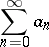(*)

is summable by Abel's method to a sumand, then the series converges to.

2) In order that summability of the series (*) by Abel's method to a sumimplies convergence of this series to this sum, it is necessary and sufficient that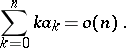Theorem 1) was later strengthened; namely, it was proved that the conditioncan be replaced by. Conditions other than summability imposed on the series are called Tauberian conditions in such cases. These conditions can be expressed in various forms. For a series (*), the most widespread conditions are of the type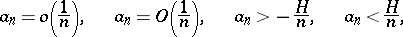whereis a constant,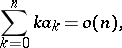and also their generalizations, in which the natural parameteris replaced by a variable. In Tauberian theorems, such conditions include, apart from those adduced above, for instance, the following one: If the series (*) is summable by Borel's method (cf. Borel summation method) to a sumand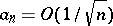, then the series converges to.

For every regular matrix summation method (cf. also Regular summation methods) there exists numbers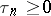such thatand the condition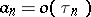is Tauberian for this method (that is, summability of the series by this method and the conditionimply convergence of the series).

Tauberian conditions can be expressed by evaluation of the partial sums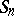of the series or by evaluation of the difference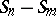with well-defined relations betweenand. Here are some examples of Tauberian theorems with such conditions: If the series (*) with partial sumsis summable by Borel's method to a sumand if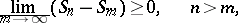with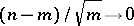, then the series converges to; if the series (*) is summable by Abel's method to a sumand its partial sumssatisfy the condition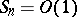, then it is summable toby the Cesàro method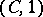(cf. Cesàro summation methods).

Lacunarity of a series,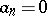when(cf. Lacunary series), can serve as a Tauberian condition; in this case, the condition is expressed in terms of properties of the sequence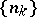.

Apart from ordinary summability, in the theory of summation Tauberian theorems are considered for special types of summability (absolute, strong, summability with a weight, etc.).